# 10 Facts about Coordinate Plane

Monday, March 14th 2016. | Mathematics

Facts about Coordinate Plane tell you about the two dimensional surface which consists of x and y coordinates. The two scales on the coordinate place are called the x-axis and y-axis. When you create the coordinate plane, you can use curves, lines or point. Here are other interesting facts about coordinate planes.

## Facts about Coordinate Plane 1: the points of the planes

There are two numbers of x and y coordinates that the people use to locate the points of the planes.

### Facts about Coordinate Plane 2: the x and y coordinates

The x and y coordinates show the horizontal and vertical distances from the origin or a specific location of the plane.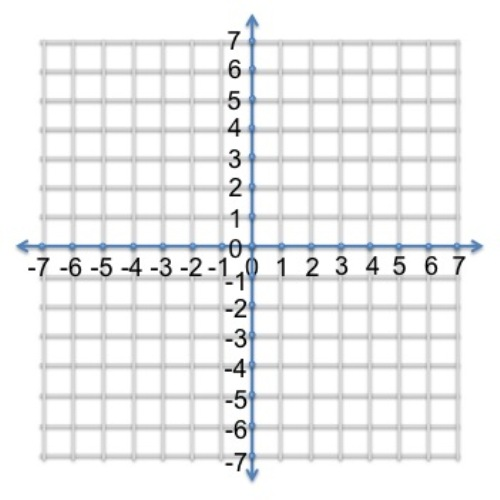Coordinate Plane Facts

## Facts about Coordinate Plane 3: what is the x-axis?

The x-axis is the horizontal scale. You can get the positive value if you move from zero to the right. If you go from the zero to the left, the value will be negative.

### Facts about Coordinate Plane 4: the y-axis

The y-axis is the vertical scale of the coordinate plane. You can get the positive direction when it goes from zero to the upper part. It will be negative if you go down from zero.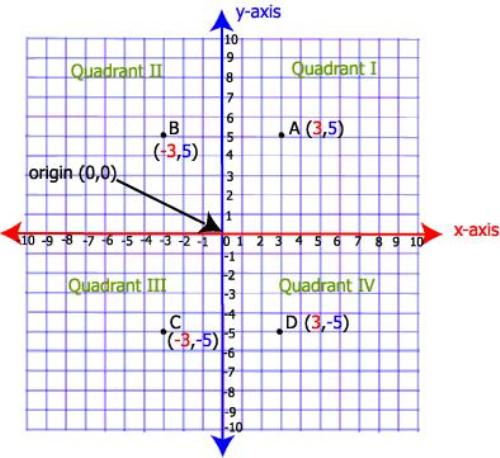Coordinate Plane Image

## Facts about Coordinate Plane 5: the origin

What is the origin? It is the point where the x and y axis is measured.

Another important term when learning coordinate plane is quadrant. The quadrant is use to call the four areas divided by the two axes in coordinate plane.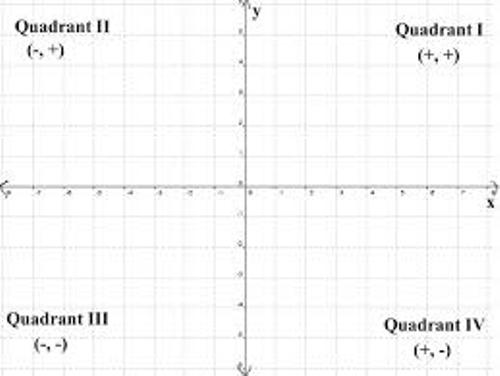Coordinate Plane Pictures

The top right of the coordinate is called the first quadrant by convention. Then you can decide the second, third and fourth quadrants in counter-clockwise direction.   In some cases, the quadrant is also labeled in I, II, III, and IV based on the Roman numerals.

### Facts about Coordinate Plane 8: René Descartes

René Descartes was the mathematician and philosopher from French who developed the Cartesian coordinate in 1637. The idea was also taken from the independent work of Pierre de Fermat. Check facts about area and perimeter here.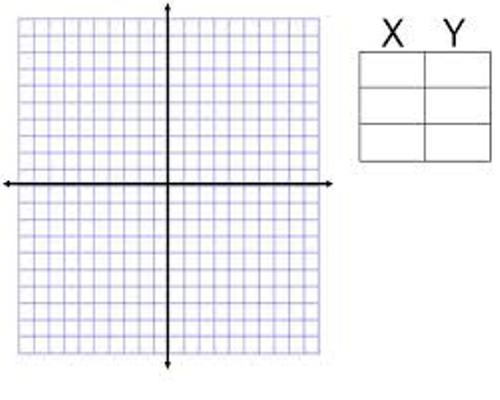## Facts about Coordinate Plane 9: the usage of a single axis

The usage of a single axis was employed by Descartes or even Fermat. The usage of pair of axes was employed after Frans van Schooten and his students translated the book of Descartes’ La Géométrie.

### Facts about Coordinate Plane 10: other coordinate systems

There are various types of coordinate systems which have been developed after the work of Descartes. Those include cylindrical coordinates and polar coordinates. Find facts about binary code here.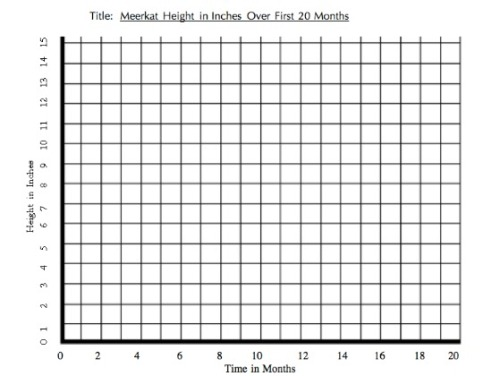Coordinate Plane Pic

Do you have any opinion on facts about coordinate plane?

tags: ,